# How To Draw a Line

Connecting the dots from default styles to textured art

## Lines in art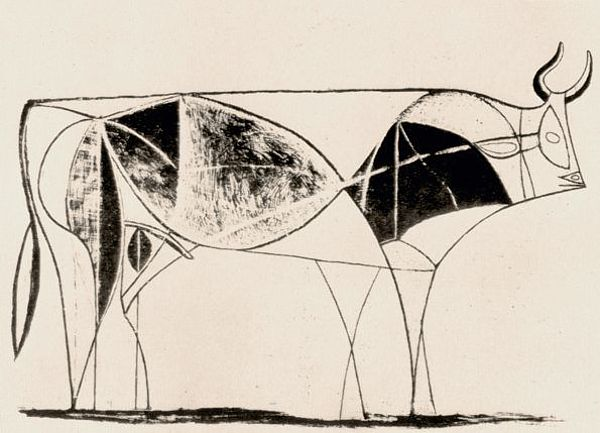Pablo Picasso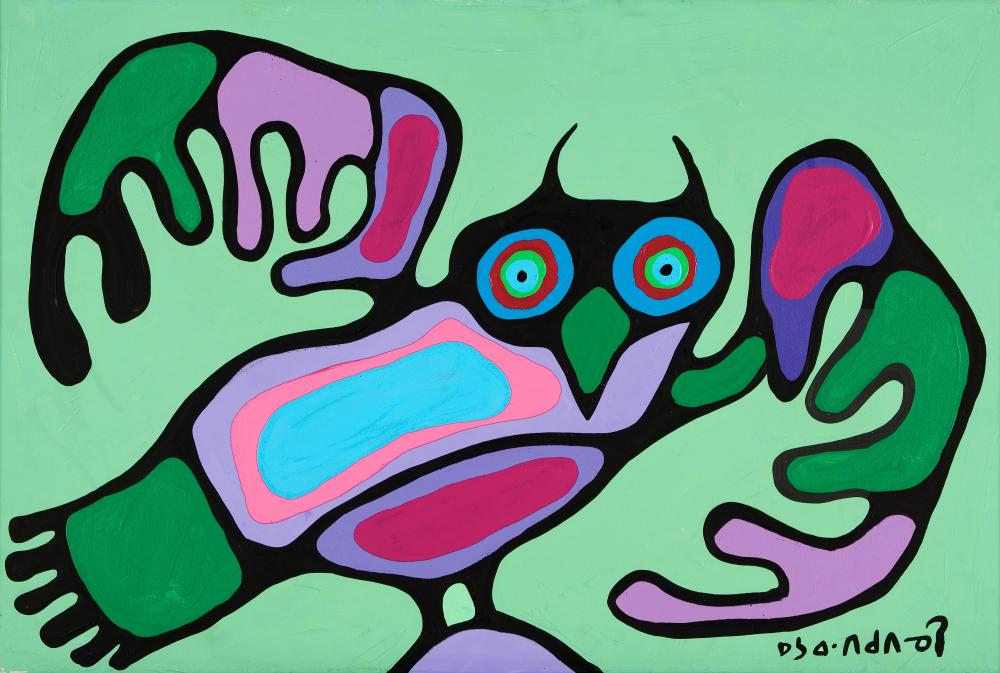Norval Morrisseau

## Lines in p5

Can we do anything differently?

## Tip: smoothing

Instead of directly adding the new point, add a point 50% of the way to the new point.

## We'll do it ourselves!

If we draw a bunch of trapezoids, we can make each segment connect

How do we get this from our points?

## Texture coordiantes

• Represents a spot on an image (between 0 and 1 on each axis)
• Use it to pin (and distort) an image to a mesh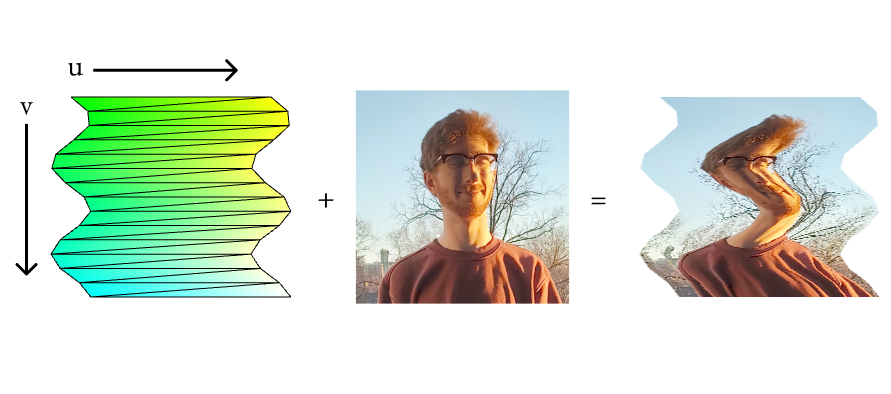• Only so much we can do with a fixed texture
• What if we could use code?
• Sounds like a job for a...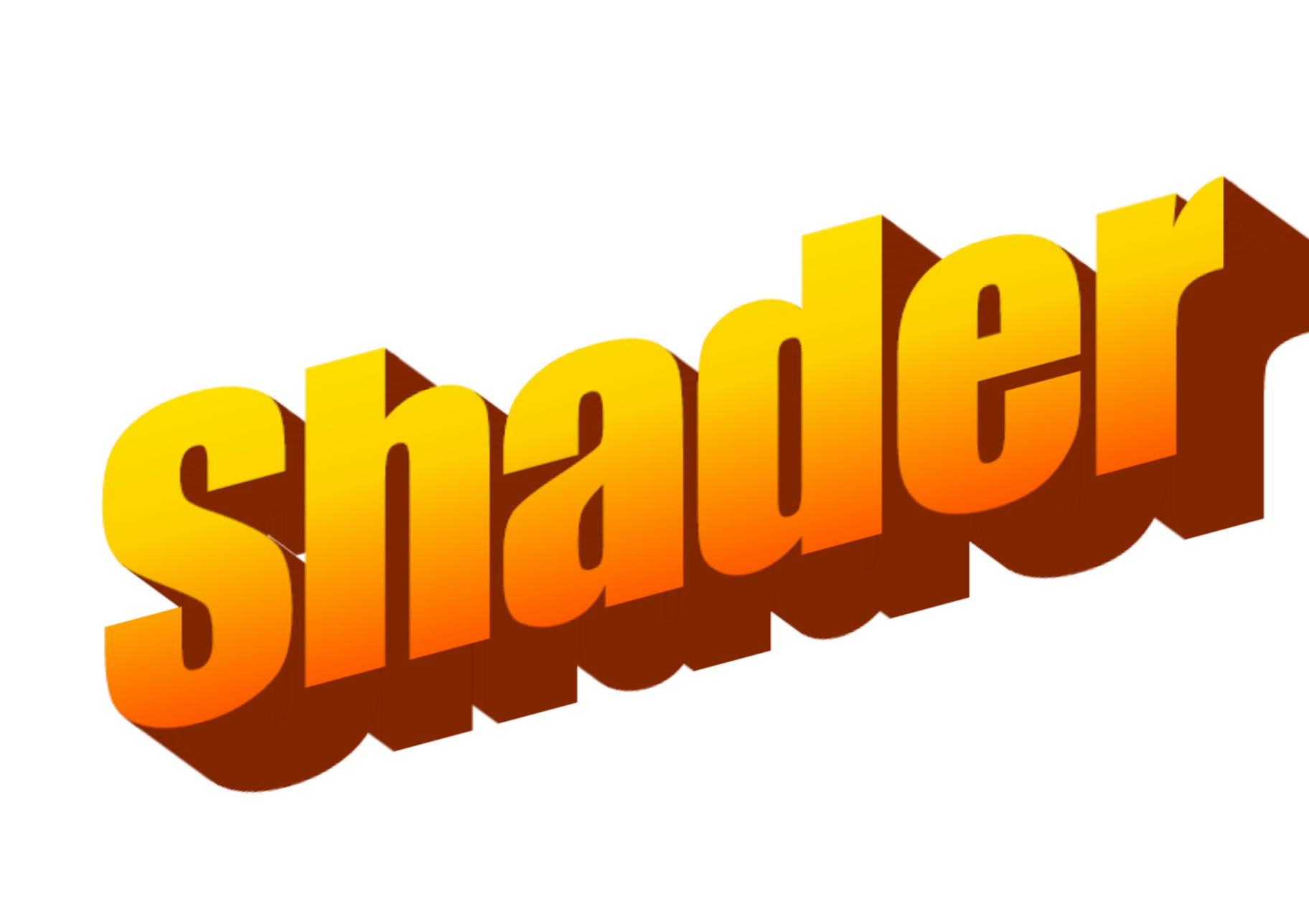Shaders are just programs that compute the position and color of shapes on your screen

Sometimes you see people online that do crazy stuff in shaders:

## Shaders don't have to be that crazy!

They're just a high-performance way of positioning shape vertices and coloring them on screen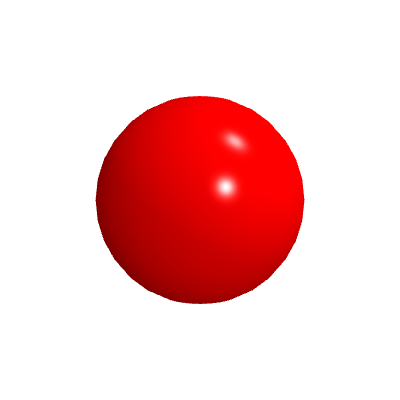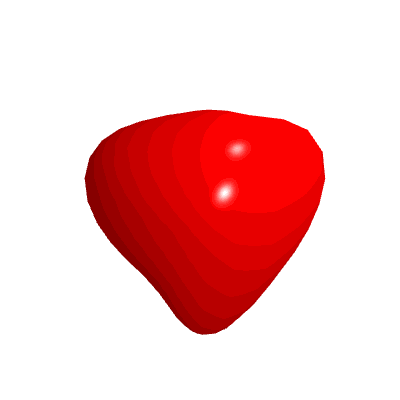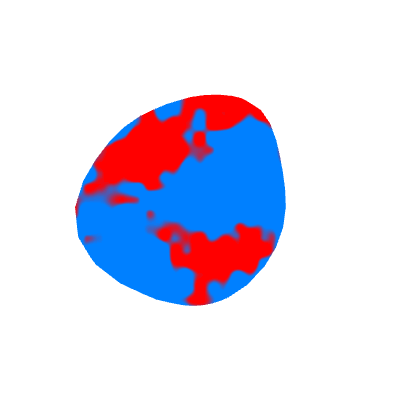## How?

WebGL gives you two spots where you can add custom rendering code via shaders:

Shape data starts in Javascript as the vertices of triangles

The vertex shader runs on each vertex in parallel calculating where on the screen it should draw

The fragment shader runs in parallel for each pixel in each triangle calculating what color it should be

## Boilerplate

```precision highp float;

attribute vec3 aPosition;
attribute vec2 aTexCoord;
attribute vec4 aVertexColor;

uniform mat4 uModelViewMatrix;
uniform mat4 uProjectionMatrix;

varying vec2 vTexCoord;
varying vec4 vVertexColor;

void main() {
// Apply the camera transform
vec4 viewModelPosition =
uModelViewMatrix *
vec4(aPosition, 1.0);

// Tell WebGL where the vertex goes
gl_Position =
uProjectionMatrix *
viewModelPosition;

// Pass along data to the fragment shader
vTexCoord = aTexCoord;
vVertexColor = aVertexColor;
}```

```precision highp float;

varying vec2 vTexCoord;
varying vec4 vVertexColor;

void main() {
// Tell WebGL what color to make the pixel
gl_FragColor = vVertexColor;
}```

## Useful functions for GLSL: noise

Courtesy of Patricio Gonzalez Vivo from The Book of Shaders

```float rand(vec2 n) {
return fract(sin(dot(n, vec2(12.9898, 4.1414))) * 43758.5453);
}

float rand(float n){return fract(sin(n) * 43758.5453123);}

float noise(float p){
float fl = floor(p);
float fc = fract(p);
return mix(rand(fl), rand(fl + 1.0), fc);
}

float noise(vec2 n) {
const vec2 d = vec2(0.0, 1.0);
vec2 b = floor(n), f = smoothstep(vec2(0.0), vec2(1.0), fract(n));
return mix(mix(rand(b), rand(b + d.yx), f.x), mix(rand(b + d.xy), rand(b + d.yy), f.x), f.y);
}```